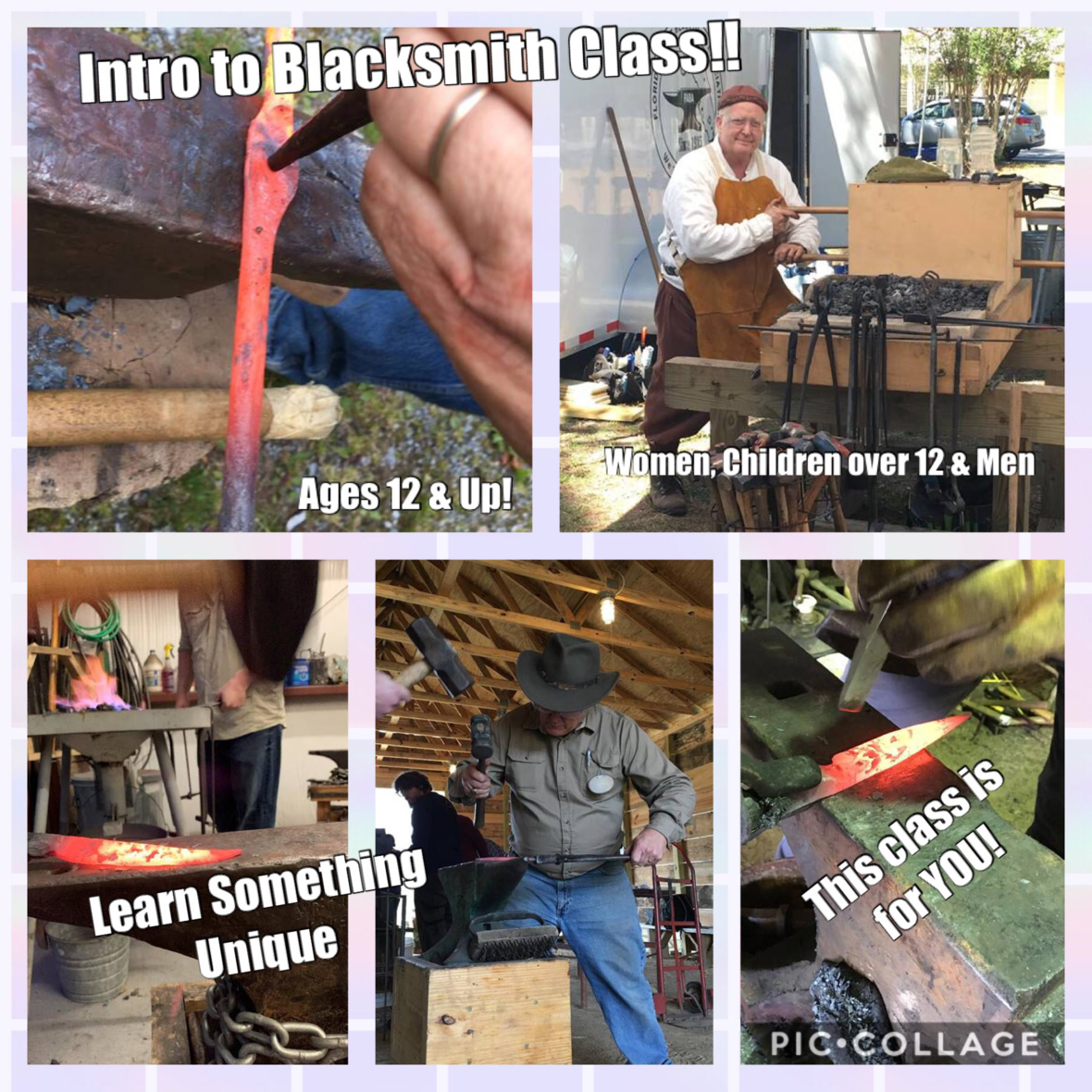Like   Tweet

## SONSHINE FAMILY FARMS/* styles */ We're giving away 6 passes to Sonshine Family Farms! Activities include - The areas biggest corn maze...8.5 acres big! Hayride, zip lines, kiddie train, mini golf, pumpkin patch, fish pond (bring your own pole), playground, corn toss, tether ball, and more! You can even stroll through their huge pumpkin patch! Check them out at Sonshine Family Farms
 table div table+table+table+table+table+table+table+table div table{width:100%;padding:0}table div table+table+table+table+table+table+table+table div table img{width:96.23%;padding:0;float:none}table div table+table+table+table+table+table+table+table div table td{width:100%;padding:0 1.88% 18px}/* styles */# OUR SECOND GIVEAWAY IS FOR AGES 12 & Up!/* styles */ INTRODUCTION TO BLACKSMITHING CLASS We're giving away an Introduction to Blacksmithing Class from Traditions Workshop. They have catered to men, women, boys and girls and we hear that women actually do great because they let the hammer do the work and strike with speed and accuracy, the two key elements for successful forging. So this very unique class is up for grabs and we're excited to offer this! Check them out at Traditions Workshop
 /* styles */ WINNERS FOR BOTH CONTESTS WILL BE DRAWN NEXT WEEK AND NOTIFIED BY EMAIL.
 table div table+table+table+table+table+table+table+table+table+table+table+table+table+table div table{width:100%;padding:0}table div table+table+table+table+table+table+table+table+table+table+table+table+table+table div table img{width:96.23%;padding:0;float:none}table div table+table+table+table+table+table+table+table+table+table+table+table+table+table div table td{width:100%;padding:0 1.88% 18px}/* styles */table div table+table+table+table+table+table+table+table+table+table+table+table+table+table+table+table div table{width:100%;padding:0}table div table+table+table+table+table+table+table+table+table+table+table+table+table+table+table+table div table img{width:96.23%;padding:0;float:none}table div table+table+table+table+table+table+table+table+table+table+table+table+table+table+table+table div table td{width:100%;padding:0 1.88% 18px}/* styles */table.module-17{width:98.49%;padding:0}table div table+table+table+table+table+table+table+table+table+table+table+table+table+table+table+table+table+table div table{width:98.49%;float:none;margin-left:auto;margin-right:auto;padding:0}table div table+table+table+table+table+table+table+table+table+table+table+table+table+table+table+table+table+table div table a{border:0 none;text-decoration:none}table div table+table+table+table+table+table+table+table+table+table+table+table+table+table+table+table+table+table div table img{width:100%!important;border:0 none;text-decoration:none}table div table+table+table+table+table+table+table+table+table+table+table+table+table+table+table+table+table+table div table td{width:100%;padding:0}/* styles */Art Parties for All! We Come to YOU!

## LOWER or ELIMINATE Your Electricity Bill

 table.module-21{width:96.6%;padding:0}table div table+table+table+table+table+table+table+table+table+table+table+table+table+table+table+table+table+table+table+table+table+table div table{width:96.6%;float:none;margin-left:auto;margin-right:auto;padding:0}table div table+table+table+table+table+table+table+table+table+table+table+table+table+table+table+table+table+table+table+table+table+table div table a{border:0 none;text-decoration:none}table div table+table+table+table+table+table+table+table+table+table+table+table+table+table+table+table+table+table+table+table+table+table div table img{width:100%!important;border:0 none;text-decoration:none}table div table+table+table+table+table+table+table+table+table+table+table+table+table+table+table+table+table+table+table+table+table+table div table td{width:100%;padding:0}/* styles */